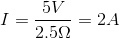# MCAT Physical : Resistivity

## Example Questions

### Example Question #2 : Resistivity

Which of the following factors would decrease the resistance through an electrical cord?

Increasing the length of the cord

Decreasing the cross-sectional area of the cord

Increasing the cross-sectional area of the cord

Increasing the resistivity

Increasing the cross-sectional area of the cord

Explanation:

The equation for resistance is given by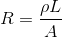.

From this equation, we can see the best way to decrease resistance is by increasing the cross-sectional area,, of the cord. Increasing the length,, of the cord or the resistivity,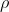, will increase the resistance.

### Example Question #3 : Resistivity

An electrician wishes to cut a copper wire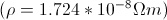that has no more than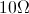of resistance. The wire has a radius of 0.725mm. Approximately what length of wire has a resistance equal to the maximum?

2.6cm

9.6m

960m

10cm

38m

960m

Explanation:

To relate resistance R, resistivity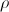, area A, and length L we use the equation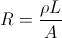.

Rearranging to isolate the quantity we wish to solve for, L, gives the equation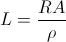. We must first solve for A using the radius, 0.725mm.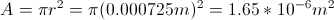Plugging in our numbers gives the answer, 960m.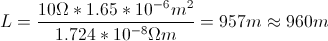### Example Question #61 : Circuits

What is the current in a circuit with a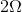resistor followed by a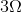resistor that are both in parallel with a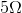resistor? The voltage supplied to the circuit is 5V.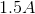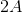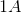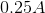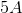Explanation:

Resistors in serries add according to the formula: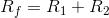Resistors in parallel add according to the formula: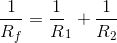We can find the total equivalent resistance: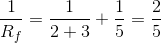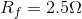Now we can use Ohm's law to find the current: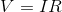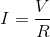Solve: